CBSE Class 12 Sample Paper for 2022 Boards (MCQ Based - for Term 1)

Class 12
Solutions of Sample Papers and Past Year Papers - for Class 12 Boards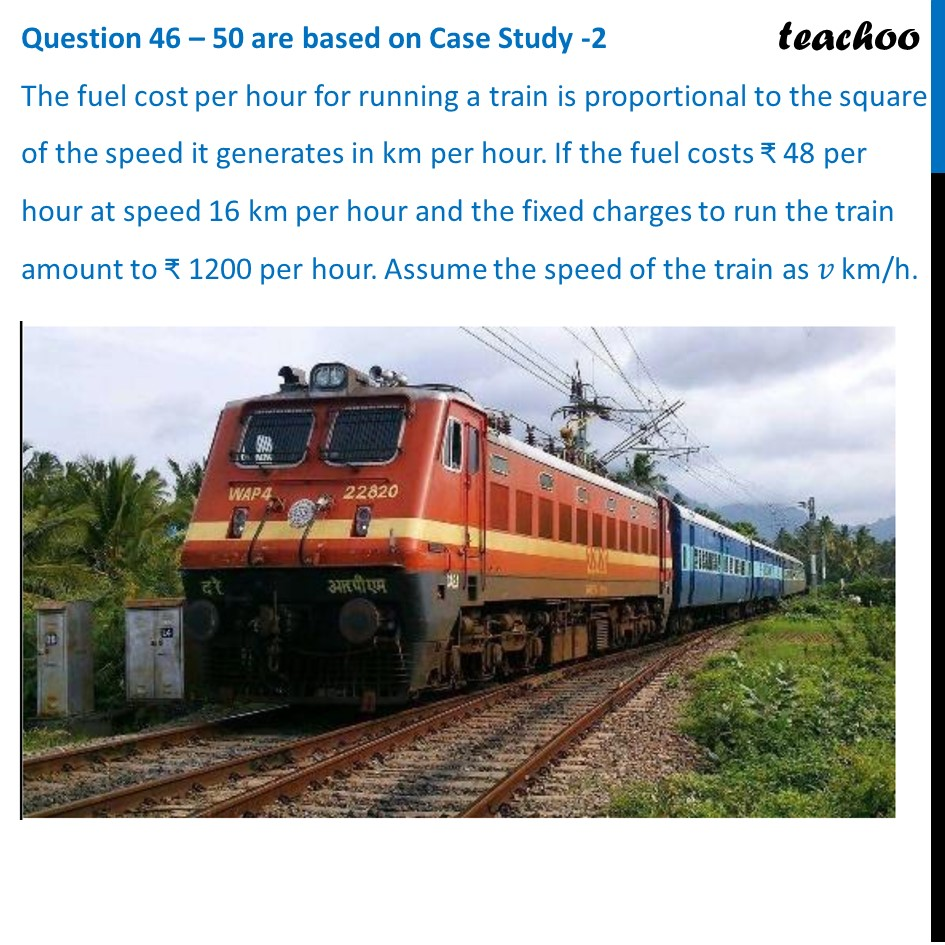## The fuel cost per hour for running a train is proportional to the square of the speed it generates in km per hour. If the fuel costs ₹ 48 per hour at speed 16 km per hour and the fixed charges to run the train amount to ₹ 1200 per hour. Assume the speed of the train as 𝑣 km/h.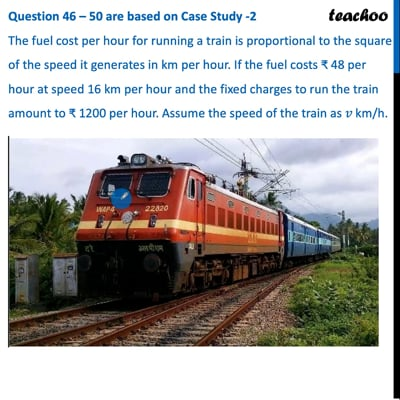This video is only available for Teachoo black users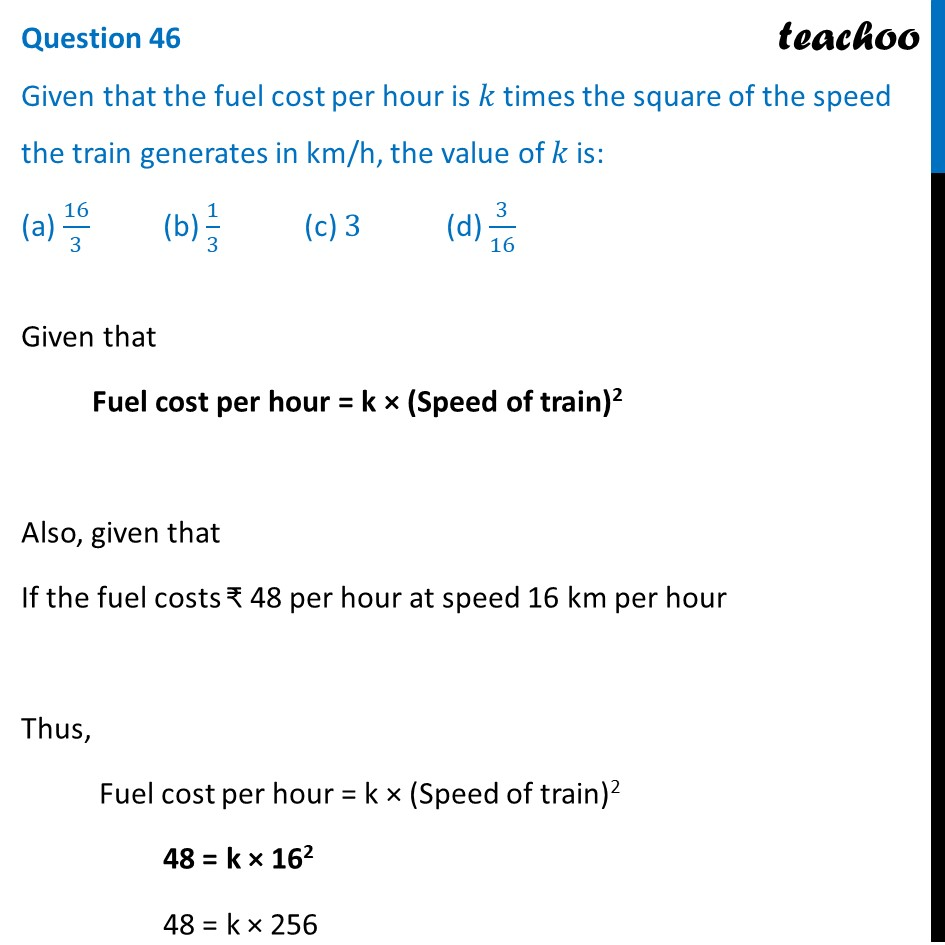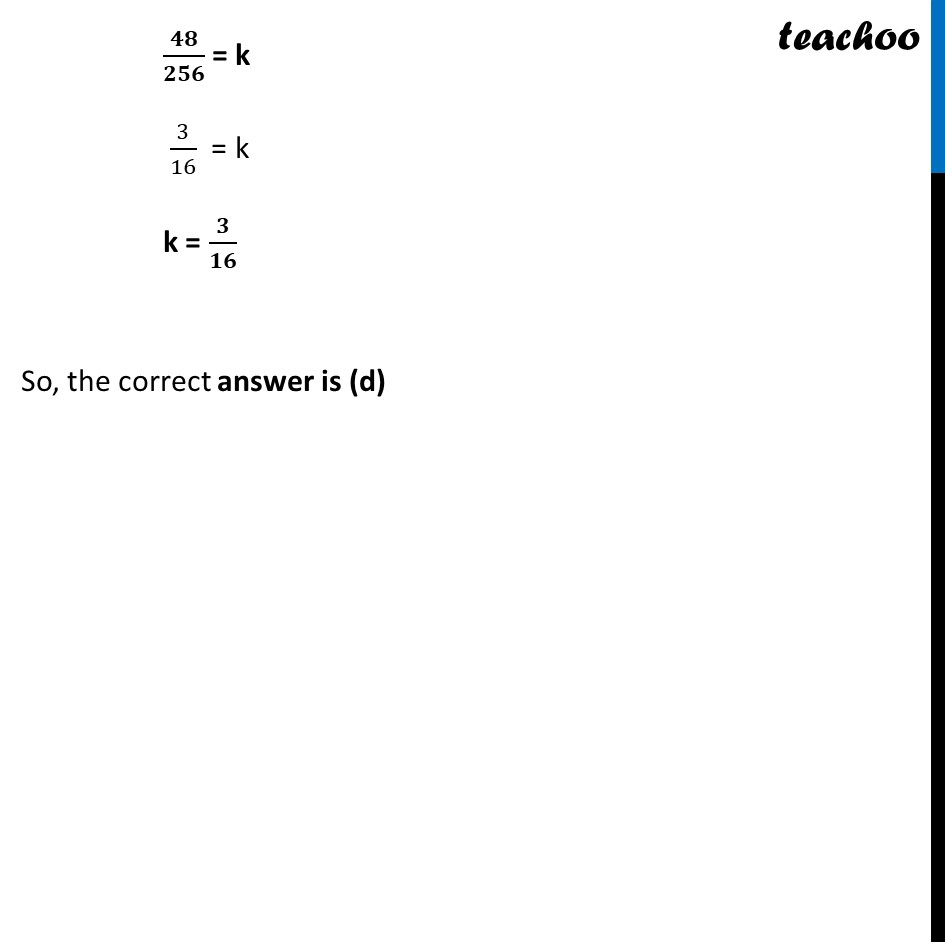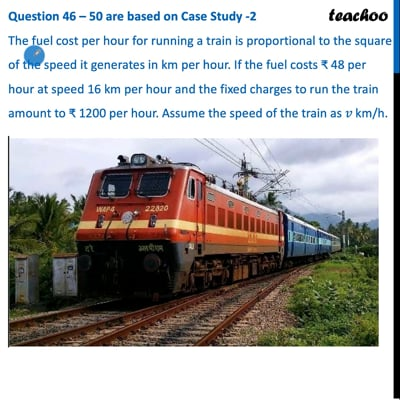This video is only available for Teachoo black users

Learn in your speed, with individual attention - Teachoo Maths 1-on-1 Class

### Transcript

Question 46 – 50 are based on Case Study -2 The fuel cost per hour for running a train is proportional to the square of the speed it generates in km per hour. If the fuel costs ₹ 48 per hour at speed 16 km per hour and the fixed charges to run the train amount to ₹ 1200 per hour. Assume the speed of the train as 𝑣 km/h. Question 46 Given that the fuel cost per hour is 𝑘 times the square of the speed the train generates in km/h, the value of 𝑘 is: (a) 16/3 (b) 1/3 (c) 3 (d) 3/16 Given that Fuel cost per hour = k × (Speed of train)2 Also, given that If the fuel costs ₹ 48 per hour at speed 16 km per hour Thus, Fuel cost per hour = k × (Speed of train)2 48 = k × 162 48 = k × 256 𝟒𝟖/𝟐𝟓𝟔 = k 3/16 = k k = 𝟑/𝟏𝟔 So, the correct answer is (d)